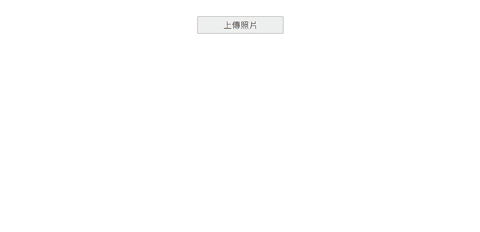#DAY 25
1
Modern Web

## [重構倒數第06天] - 前端除了要做預覽圖還要把圖片變模糊 !

### 前言``````import { usePhotoToBlur } from "../composition-api/usePhotoToBlur.js";

// 其他省略...

async function useQueuePreview(fileArr) {
const { parsePhoto } = usePhotoToBlur();

// 多圖多影片列表
const previewMap = {};

// 排序索引
let idx = 0;

for (const file of fileArr) {
const blob = useImageFilePreview(file);
const blur = await parsePhoto(file);
previewMap[idx] = {
id: idx,
src: blob,
blurred_url: blur
};
idx++;
}

return previewMap;
}

// 其他省略...

``````

`usePhotoToBlur.js`

``````export function usePhotoToBlur() {
const parsePhoto = (imgUrl) => {
return new Promise((resolve, reject) => {
const canvas = document.createElement("canvas");
const ctx = canvas.getContext("2d");
try {
const img = new Image();
canvas.width = img.width;
canvas.height = img.height;
ctx.drawImage(img, 0, 0);
const base64 = gBlur(6, canvas, ctx);
resolve(base64);
};
img.src = event.target.result;
};
} catch (error) {
reject(error);
}
});
};

return {
parsePhoto
};
}

``````

``````const base64 = gBlur(6, canvas, ctx);
``````

``````const gBlur = (blur = 8, h5canvas, context) => {
const canvas = h5canvas;
const ctx = context;
let sum = 0;
let delta = 5;
let alpha_left = 1 / (2 * Math.PI * delta * delta);
let step = blur < 3 ? 1 : 2;
for (let y = -blur; y <= blur; y += step) {
for (let x = -blur; x <= blur; x += step) {
let weight =
alpha_left * Math.exp(-(x * x + y * y) / (2 * delta * delta));
sum += weight;
}
}
for (let y = -blur; y <= blur; y += step) {
for (let x = -blur; x <= blur; x += step) {
ctx.globalAlpha =
((alpha_left * Math.exp(-(x * x + y * y) / (2 * delta * delta))) /
sum) *
blur;
ctx.drawImage(canvas, x, y);
}
}
ctx.globalAlpha = 1;
const base64 = canvas.toDataURL();
return base64;
};
``````

``````// 其他省略...
async function useQueuePreview(fileArr) {
const { parsePhoto } = usePhotoToBlur();
const previewMap = {};
let idx = 0;
for (const file of fileArr) {
const blob = useImageFilePreview(file);

// 等待模糊處理完後，在執行後續的動作
const blur = await parsePhoto(file);

previewMap[idx] = {
id: idx,
src: blob,
blurred_url: blur
};
idx++;
}
return previewMap;
}

export function useFileUpdate() {
const previewMap = ref({});
const initData = () => {
previewMap.value = {};
};

const setFile = async (file = []) => {
initData();
// 這邊也是需要等到 useQueuePreview 都處理完後在把資料寫回 previewMap
previewMap.value = await useQueuePreview(file);
};

return { setFile, previewMap };
}
``````

``````  <div
v-show="Object.values(previewMap).length !== 0"
class="img_box"
v-for="item in previewMap"
:key="item.id"
:style="{ 'background-image': `url(\${item.blurred_url})` }"
>
<img :src="item.src" alt="" />
</div>
``````### 最後### 那如果對於Vue3不夠熟的話呢?

Ps. 購買的時候請登入或註冊該平台的會員，然後再使用下面連結進入網站點擊「立即購課」，這樣才可以讓我獲得更多的課程分潤，還可以幫助我完成更多豐富的內容給各位。

https://hiskio.com/packages/AYR5m7VR3

https://hiskio.com/packages/Q9R4OYoyD